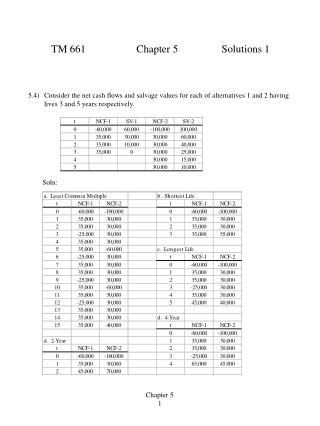DownloadDownload PresentationTM 661 Chapter 5 Solutions 1

TM 661 Chapter 5 Solutions 1

Télécharger la présentationTM 661 Chapter 5 Solutions 1

- - - - - - - - - - - - - - - - - - - - - - - - - - - E N D - - - - - - - - - - - - - - - - - - - - - - - - - - -
Presentation Transcript

1. TM 661 Chapter 5 Solutions 1 5.4) Consider the net cash flows and salvage values for each of alternatives 1 and 2 having lives 3 and 5 years respectively. Soln: Chapter 5 1

2. TM 661 Chapter 5 Solutions 2 5-10) A firm has available 4 proposals A, B, C, D. Proposal is contingent on acceptance of either proposal C or proposal D. In addition, proposal C is contingent on proposal D, while proposal D s contingent on either proposal A or proposal B. The firm has a budget limitation of \$500,000. The cash flows are as follows: Initial Costs A = 250,000 B = 350,000 C = 50,000 D = 38,000 Soln: Note we did not need to include the entire cash flow since only the initial costs affect the initial budget of \$500,000. Alternative Acceptable Reason A, B, C, D no exceeds budget A, B, C no exceeds budget A, B, D no exceeds budget A, C, D yes A, C no C contingent on D A, B no exceeds budget A, D yes B, C, D yes B, C no C is contingent on D B, D yes C, D no D is contingent on A or B A no A is contingent on C or D B yes C no C is contingent on D D no D is contingent on A or B Chapter 5 2

3. TM 661 Chapter 5 Solutions 3 5-23) Two mutually exclusive proposals, each with a life of 5 years, are under consideration. MARR is 12%. Each proposal has the following cash flow profile. Soln: a. Net Present Worth A NPW = -30,000 + 9,300 (P/A, 12, 5) = -30,000 + 9,300 (3.6048) = \$ 3,525 B NPW = -42,000 + 12,625 (3.6048) = \$3,511 Choose A b. Incremental Method NPW = -12,000 + 3,325(3.6048) = -14 Choose A Chapter 5 3

4. TM 661 Chapter 5 Solutions 3 5-23) (Cont) Soln: c. Equivalent Annual Worth A EUAW = -30,000 (A/P, 12, 5) + 9,300 = -30,000 (0.2774) + 9,300 = \$ 978 B EUAW = -42,000 (0.2774) + 12,625 = \$ 974 Choose A Chapter 5 4

5. TM 661 Chapter 5 Solutions 5 5-36) An aluminum extrusion plant manufactures a particular product at a variable cost of \$0.04 per unit, including material costs. The fixed costs associated with manufacturing the product equal \$30,000 per year. Determine the break-even value for annual sales if the selling price per unit is a) \$0.40, b) \$0.30, and c) \$0.20. Soln: Let S = selling price Profit = SX - 30,000 - .04X = (S - .04)X -30,000 Since profit = 0 at breakeven X = 30,000 / (S - .04) a. X = 30,000 / (.4 - .04) = 83,333 b. X = 30,000 / (.3 - .04) = 115,384 c. X = 30,000 / (.2 - .04) = 187,500 Chapter 5 5

6. TM 661 Chapter 5 Solutions 6 5-37) Owners of a nationwide motel chain are considering locating a new motel in Snyder, Arkansas. The complete cost of building a 150-unit motel is \$5 million. The firm estimates that the furnishings will cost \$1,875,000 initially and every 5 years thereafter. Annual operating and maintenance cost for the facility is estimated to be \$125,000. The average rate for a unit is anticipated to be \$55 per day. A 15 year planning horizon is used by the firm in evaluating new ventures of this type. Salvage value is estimated at \$1 million. Determine the breakeven if the daily occupancy is estimated to be 10%. Soln: I set up a spreadsheet showing revenues and costs. Revenues were based on an occupancy rate in cell C3. Net present worth is computed at 10%. I then just altered cell C3 until I got a NPV relatively close to 0. I compute an occupancy rate of 37.5%. Chapter 5 6